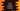# How to convert a boolean to string in Java## How to convert a boolean to string in Java :

In this quick tutorial, we will learn how to convert a boolean value to string in Java. Not only one, we have two different ways to do the conversion. Let’s take a look :

### Using Boolean.toString() :

Boolean class has one static method toString to convert a boolean value to string. The method definition is as below :

``public static String valueOf(boolean b)``
• We need to pass one boolean value as parameter to this function.
• It will return the string representation of the boolean value.

Now, let’s try it with an example :

``````class Example {
public static void main(String args[]) {
boolean firstBoolean = true;
boolean secondBoolean = false;

System.out.println("The value of firstBoolean is "+Boolean.toString(firstBoolean));
System.out.println("The value of secondBoolean is "+Boolean.toString(secondBoolean));
}
}``````

It will print the below output :

``````The value of firstBoolean is true
The value of secondBoolean is false``````

As you can see that we have two Boolean variables with true and false values. With the Boolean.toString() methods, they are converted to string “true” and “false” respectively.

### Using String.valueOf() :

Similar to the above example, we also have one static method in the String class : valueOf(). The definition of this method is as below :

``public static String valueOf(boolean b)``
• Similar to the above example, valueOf method takes one boolean as parameter and returns one string.
• It is also a static method similar to the above one.

Example of valueOf() :

``````class Example {
public static void main(String args[]) {
boolean firstBoolean = true;
boolean secondBoolean = false;

System.out.println("The value of firstBoolean is "+String.valueOf(firstBoolean));
System.out.println("The value of secondBoolean is "+String.valueOf(secondBoolean));
}
}``````

Output :

``````The value of firstBoolean is true
The value of secondBoolean is false``````